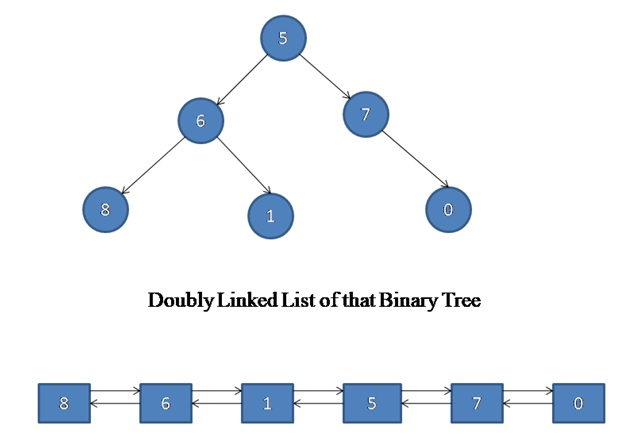# Convert a given binary Tree to Doubly Linked List (DLL)

In this article, we are going to learn how to convert a binary tree to a DLL (Doubly Linked List) using C++ program?
Submitted by Souvik Saha, on May 06, 2019

Given a Binary tree and we have to convert it to a Doubly Linked List (DLL).Algorithm:

To solve the problem we can follow this algorithm:

1. We start to convert the tree node to DLL from the rightmost tree node to the leftmost tree node.
2. Every time we connect the right pointer of a node to the head of the DLL.
3. Connect the left pointer of the DLL to that node.
5. Repeat the process from right to left most node of the tree.

C++ implementation:

```#include <bits/stdc++.h>
using namespace std;

struct node {
int data;
node* left;
node* right;
};

//Create a new node
struct node* create_node(int x)
{
struct node* temp = new node;
temp->data = x;
temp->left = NULL;
temp->right = NULL;
return temp;
}

//convert a BST to a DLL
{
if (root == NULL)
return;
}
}

//Print the list
{
while (temp) {
cout << temp->data << " ";
temp = temp->right;
}
}

//print the tree in inorder traversal
void print_tree(node* root)
{
if (root == NULL) {
return;
}
print_tree(root->left);
cout << root->data << " ";
print_tree(root->right);
}

int main()
{
struct node* root = create_node(5);
root->left = create_node(6);
root->right = create_node(7);
root->left->left = create_node(8);
root->left->right = create_node(1);
root->right->right = create_node(0);
cout << "Print Tree" << endl;
print_tree(root);
cout << "\nDoubly Linked List" << endl;

return 0;
}
```

Output

```Print Tree
8 6 1 5 7 0
8 6 1 5 7 0
```